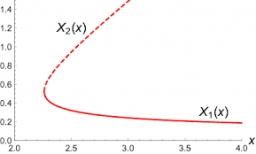# Summands 54861 ​​

Can you divide the number 64.9 into three summands so that the first with the second is in the ratio 4:5 and the third with the first in the ratio 7:3?

a =  14.16
b =  17.7
c =  33.04

### Step-by-step explanation:

a+b+c=64.9
a=4/5·b
c=7/3·a

a+b+c = 64.9
5a-4b = 0
7a-3c = 0

Pivot: Row 1 ↔ Row 3
7a-3c = 0
5a-4b = 0
a+b+c = 64.9

Row 2 - 5/7 · Row 1 → Row 2
7a-3c = 0
-4b+2.143c = 0
a+b+c = 64.9

Row 3 - 1/7 · Row 1 → Row 3
7a-3c = 0
-4b+2.143c = 0
b+1.429c = 64.9

Row 3 - 1/-4 · Row 2 → Row 3
7a-3c = 0
-4b+2.143c = 0
1.964c = 64.9

c = 64.9/1.96428571 = 33.04
b = 0-2.1428571428571c/-4 = 0-2.14285714 · 33.04/-4 = 17.7
a = 0+3c/7 = 0+3 · 33.04/7 = 14.16

a = 354/25 = 14.16
b = 177/10 = 17.7
c = 826/25 = 33.04

Our linear equations calculator calculates it.Did you find an error or inaccuracy? Feel free to write us. Thank you!

Tips for related online calculators
Check out our ratio calculator.
Do you have a system of equations and looking for calculator system of linear equations?